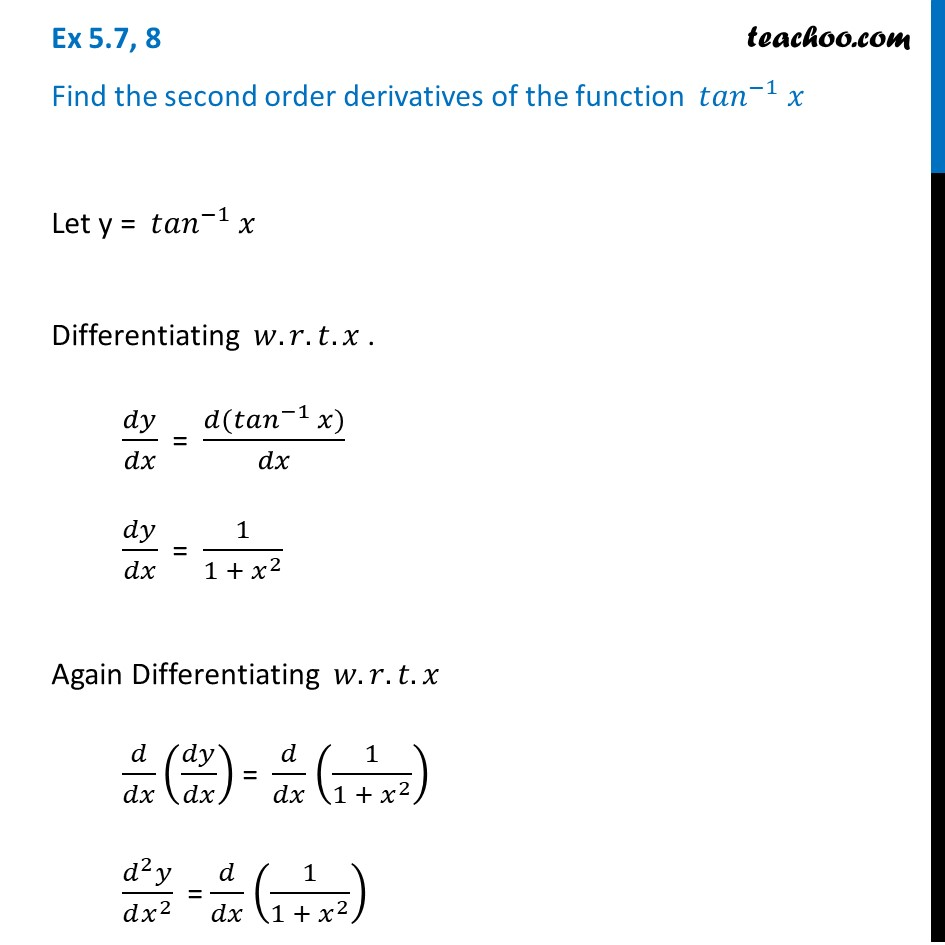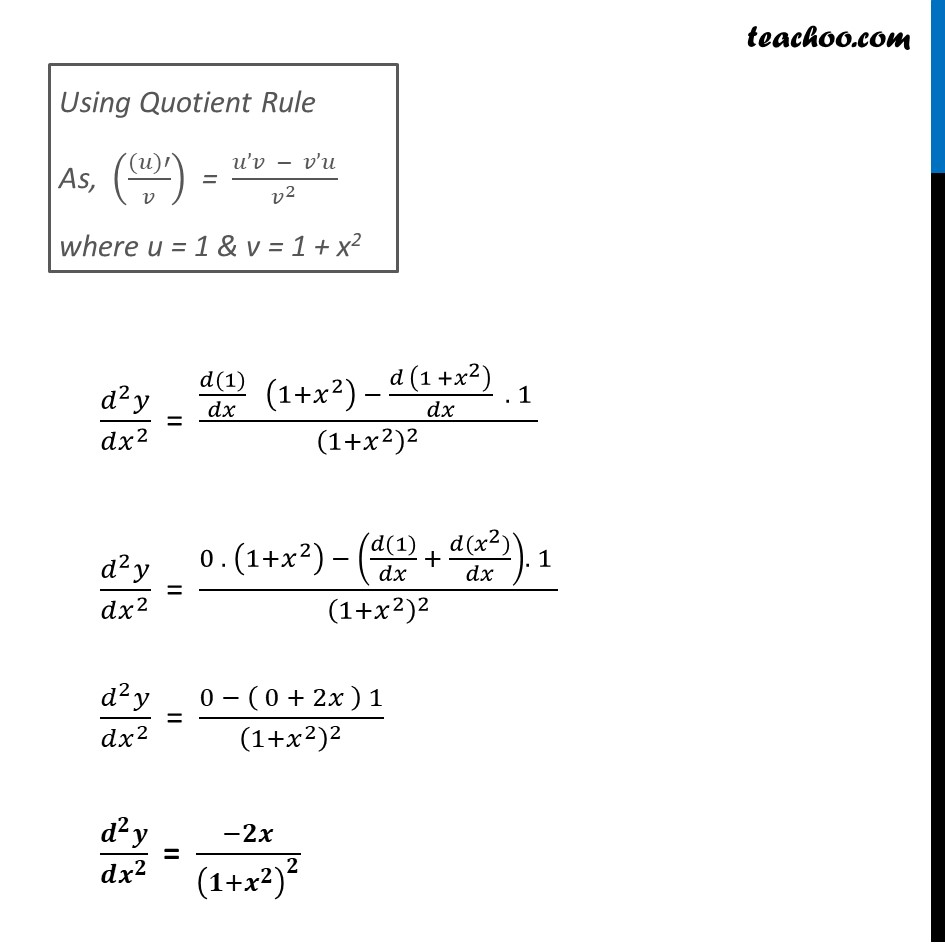Finding second order derivatives - Normal form

Chapter 5 Class 12 Continuity and Differentiability
Concept wiseIntroducing your new favourite teacher - Teachoo Black, at only ₹83 per month

### Transcript

Ex 5.7, 8 Find the second order derivatives of the function 〖𝑡𝑎𝑛〗^(−1) 𝑥 Let y = 〖𝑡𝑎𝑛〗^(−1) 𝑥 Differentiating 𝑤.𝑟.𝑡.𝑥 . 𝑑𝑦/𝑑𝑥 = (𝑑(〖𝑡𝑎𝑛〗^(−1) 𝑥))/𝑑𝑥 𝑑𝑦/𝑑𝑥 = 1/(1 + 𝑥^2 ) Again Differentiating 𝑤.𝑟.𝑡.𝑥 𝑑/𝑑𝑥 (𝑑𝑦/𝑑𝑥) = 𝑑/𝑑𝑥 (1/(1 + 𝑥^2 )) (𝑑^2 𝑦)/(𝑑𝑥^2 ) = 𝑑/𝑑𝑥 (1/(1 + 𝑥^2 )) Using Quotient Rule As, (((𝑢)′)/𝑣) = (𝑢’𝑣 − 𝑣’𝑢)/𝑣^2 where u = 1 & v = 1 + x2 (𝑑^2 𝑦)/(𝑑𝑥^2 ) = ((𝑑(1))/𝑑𝑥 (1+𝑥^2 ) − (𝑑 (1 +𝑥^2 ))/𝑑𝑥 . 1 )/(1+𝑥^2 )^2 (𝑑^2 𝑦)/(𝑑𝑥^2 ) = (0 . (1+𝑥^2 ) − ((𝑑(1))/𝑑𝑥 + (𝑑〖(𝑥〗^2))/𝑑𝑥). 1 )/(1+𝑥^2 )^2 (𝑑^2 𝑦)/(𝑑𝑥^2 ) = (0 − ( 0 + 2𝑥 ) 1)/(1+𝑥^2 )^2 (𝒅^𝟐 𝒚)/(𝒅𝒙^𝟐 ) = (−𝟐𝒙)/(𝟏+𝒙^𝟐 )^𝟐